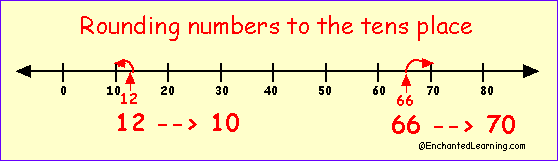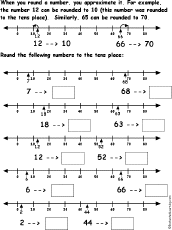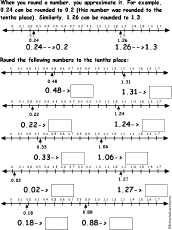EnchantedLearning.com is a user-supported site.
As a bonus, site members have access to a banner-ad-free version of the site, with print-friendly pages.

 More Math EnchantedLearning.comRounding NumbersInformation and Printable Worksheets Place Value

When a number is rounded (or rounded off), it is approximated by eliminating the least significant digits. When rounding, you are finding the closest multiple of ten (or one hundred, or other place value) to your number. For example, the number 42 can be rounded down to 40 (this number was rounded to the tens place). Similarly, 285 can be rounded up to 300 (this number was rounded to the hundreds place).

Rounding is used to make a number easier to work with. For example, if you know that there are 496 students in your school, you can say that there are approximately 500 students in your school.On a number line, you can see how rounding a number approximates its value.

Whole numbers can be rounded to the tens place, hundreds place, thousands place, and so on. When a number is rounded to the tens place, the final form has a zero for the ones digit. When a number is rounded to the hundreds place, the final form has a zero for both the tens digit and the ones digit.

Decimal numbers can also be rounded; this approximates the number to the nearest tenth, hundredth, thousandth, or other decimal place. When a decimal number is rounded to the tenths place, the final form has no digit in the hundredths place (or any places to the right of that). When a decimal number is rounded to the hundredths place, the final form has no digit in the thousandths place (or any places to the right of that).

How to Round Off a Number:
 To round off a number, the digit to be rounded either remains the same or is increased by one, and one or more of the digits on the right are eliminated or changed to zero. In order to round a number, do the following: Determine which digit is to be rounded - now look at the digit to the right of this.For example, if you are rounding the number 246 to the tens digit, the digit "4" (the tens digit) will be rounded and the "6" (the ones digit) is the digit that will become zero. If the digit to become zero is 0, 1, 2, 3, or 4, that digit becomes a zero (as do all the digits to the right of it) and the digit to be rounded (the digit immediately to the left of it) remains the same. If the digit to become zero is 6, 7, 8 or 9, that digit becomes a zero (as do all the digits to the right of it) and the digit to be rounded is increased by one.In our example of rounding 246 to the tens digit, the "6" is large enough to make the next digit increase by one (from "4" to "5"), and the number is rounded to 250. If the digit to become zero is 5: If the digit to the right of the digit is to be rounded is a five (like 15 rounded to the tens digit or 257 rounded to the hundreds digit), then there are two possibilities. If there is at least one non-zero digit after the five, then the digit to be rounded increases by one and the five becomes a zero (as do all the remaining digits). For example, 257 rounded to the hundreds digit becomes 300, and 1521 rounded to the thousands place becomes 2000.  If there are no digits after the five (or the digits after the five are all zeros), then the number could equally well be rounded up or down. Mathematicians have devised a rule so that in large groups of data, half the time 5s are rounded up and half the time 5s are rounded down, producing no bias in the data. In this second case, the five becomes a zero, and the number to be rounded is either left alone (if the number was originally even) or increased by one (if the number was originally odd). The rounded digit is always even. For example, 5 rounded to the tens digit is 0, 15 rounded to the tens digit is 20, and 185 rounded to the tens digit is 180.

Rounding can be used to make numbers easier to work with (and sometimes it is a necessity). For example, if 12 out of 107 people vote for candidate "C", you can quickly estimate the percent of votes for "C" to be roughly 10 out of 100 people or 1/10 of the people.

Examples of Rounding numbers:
Number Nearest ten Nearest hundred Nearest thousand
368 370 400 0
1,234 1,230 1,200 1,000
89,355 89,360 89,400 89,000

Decimal numbers can also be rounded, estimating the number to the nearest tenth, hundredth, thousandth, and so on.

Examples of Rounding Decimals:
Number Nearest tenth Nearest hundredth Nearest thousandth
1.2346 1.2 1.23 1.235
31.6479 31.6 31.65 31.648
9.7462 9.7 9.75 9.746

Worksheets on Rounding Numbers:Round Numbers to the Nearest Tensusing a number lineRound off 10 numbers to the nearest tens digit using a number line. Or go to the answers.Round Numbers to the Nearest Hundredsusing a number lineRound off 10 numbers to the nearest hundreds digit using a number line. Or go to the answers.Round Numbers to the Nearest Tens, Hundreds, or Thousands DigitRound off the numbers to the nearest tens digit, hundreds digit, or thousands digit. Worksheet #1 or go to the answersWorksheet #2 or go to the answersWorksheet #3 or go to the answers.Round Numbers to the Nearest Tens, Hundreds, and Thousands Digit in a GridOn a grid, round off the numbers to the nearest tens digit, hundreds digit, and thousands digit. Worksheet #1 or go to the answers Worksheet #2 or go to the answers Worksheet #3 or go to the answersRound Decimal Numbers to the Nearest Tenthsusing a number lineRound off 10 decimal numbers to the nearest tenths digit using a number line. Or go to the answers.Round Decimal Numbers to the Nearest Tenth, Hundredth, or Thousandth DigitRound off the numbers to the nearest tenth digit, hundredth digit, or thousandth digit. Worksheet #1 or go to the answersWorksheet #2 or go to the answersWorksheet #3 or go to the answers.Round Decimal Numbers to the Nearest Tenths, Hundredths, and Thousandths Digit in a GridOn a grid, round off the decimal numbers to the nearest tenths digit, hundredths digit, and thousandths digit. Worksheet #1 or go to the answers Worksheet #2 or go to the answers Worksheet #3 or go to the answers

 +, - EnchantedLearning.comMath x, ÷
 A B C D E F G H I J K L M N O P Q R S T U V W X Y Z
 Number Line Fractions Decimals Measurement Rounding Graphs

Enchanted Learning®
Over 35,000 Web Pages
Sample Pages for Prospective Subscribers, or click below

 Overview of Site What's New Enchanted Learning Home Monthly Activity Calendar Books to Print Site Index K-3 Crafts K-3 Themes Little ExplorersPicture dictionary PreK/K Activities Rebus Rhymes Stories Writing Cloze Activities Essay Topics Newspaper Writing Activities Parts of Speech Fiction The Test of Time Biology Animal Printouts Biology Label Printouts Biomes Birds Butterflies Dinosaurs Food Chain Human Anatomy Mammals Plants Rainforests Sharks Whales Physical Sciences: K-12 Astronomy The Earth Geology Hurricanes Landforms Oceans Tsunami Volcano Languages Dutch French German Italian Japanese (Romaji) Portuguese Spanish Swedish Geography/History Explorers Flags Geography Inventors US History Other Topics Art and Artists Calendars College Finder Crafts Graphic Organizers Label Me! Printouts Math Music Word Wheels

## Enchanted Learning Search

 Search the Enchanted Learning website for: